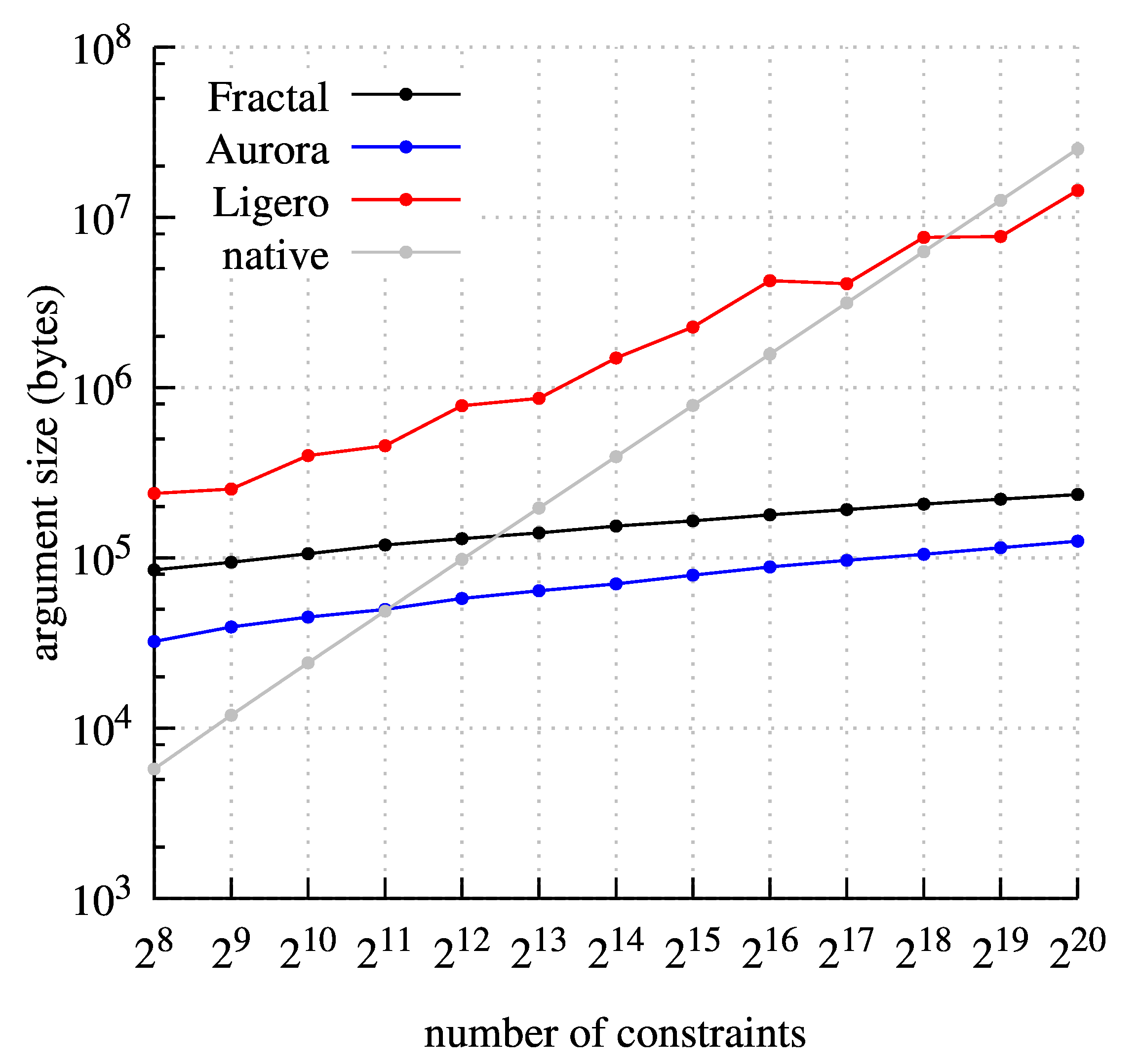{{ message }}

# scipr-lab / libiop

C++ library for IOP-based zkSNARKs

## Files

Failed to load latest commit information.
Type
Name
Commit time

# libiop: a C++ library for IOP-based zkSNARKs

This library provides zkSNARK constructions that are transparent and post-quantum, and moreover rely only on lightweight symmetric cryptography (any cryptographic hash function).

The library provides a tool chain for transforming certain types of probabilistic proofs (see below) into zkSNARKs with the above properties. The library includes several zkSNARK constructions that follow this paradigm:

• The Ligero protocol from [AHIV], whose argument size is O(N0.5).
• The Aurora protocol from [BCRSVW], whose argument size is O(log2 N).
• The Fractal protocol from [COS], whose argument size is O(log2 N).

All of these zkSNARKs support R1CS (an NP-complete relation that generalizes arithmetic circuit satisfiability) over smooth prime fields and binary extension fields. An important component of Aurora and Fractal, which is of independent interest, is the FRI low-degree test.

WARNING: This is an academic proof-of-concept prototype, and in particular has not received careful code review.
This implementation is NOT ready for production use.

## From IOPs to zkSNARKs

Interactive oracle proofs (IOPs) are a multi-round generalization of probabilistically checkable proofs (PCPs) that offer better performance compared to PCPs. This library provides zkSNARKs constructed from IOPs via the BCS transformation.

The BCS transformation uses a cryptographic hash function (modeled as a random oracle) to compile any public-coin IOP into a SNARG that is:

• transparent (the only global parameter needed to produce/validate proof strings is the hash function);
• post-quantum (it is secure in the quantum random oracle model);
• lightweight (no cryptography beyond the hash function is used).

The BCS transformation is described in [BCS], and its post-quantum security is proved in [CMS].

The BCS transformation preserves proof of knowledge: if the underlying IOP is a proof of knowledge, then the resulting SNARG is an argument of knowledge (i.e., a SNARK). Similarly, the BCS transformation preserves zero knowledge: if the underlying IOP is (honest-verifier) zero knowledge then the resulting SNARG is zero knowledge (i.e., a zkSNARG).

The BCS transformation also extends to compile holographic IOPs into preprocessing SNARGs, as described in [COS]. This feature enables SNARGs that provide fast verification for arbitrary computations (and not just structured computations).

## IOP protocols

The folder `libiop/iop` contains infrastructure for writing IOP protocols.

The folder `libiop/protocols` contains several protocols written using this infrastructure. These include:

language round
complexity
oracle length
(field elts)
query
complexity
indexer time
(field ops)
prover time
(field ops)
verifier time
(field ops)
Ligero-IOP R1CS 2 O(N) O(N0.5) N/A O(N logN) O(N)
Aurora-IOP R1CS O(log N) O(N) O(log N) N/A O(N logN) O(N)
Fractal-IOP R1CS O(log N) O(N) O(log N) O(N logN) O(N logN) O(log N)

The first is an IOP from the Ligero paper, the second is an IOP from the Aurora paper, and the third is an IOP from the Fractal paper.

Efficient IOP protocols such as the above are obtained by combining two components: (1) RS-encoded IOP, and a (2) proximity test for the RS code. (See this paper for more details.) The codebase in this library provides infrastructure that enables generically composing these components.

: More precisely, the Ligero paper only describes a construction for arithmetic circuits. An appendix of the Aurora paper explains how to extend the construction to support R1CS. The latter is the implemented protocol.

## BCS transformation

The folder `libiop/bcs` contains the BCS transformation as a standalone component.

The folder `libiop/snark` contains zkSNARKs obtained by applying the BCS transformation to the IOP protocols above.

language indexer time prover time argument size verifier time
Ligero-SNARK R1CS N/A Oκ(N logN) Oκ(N0.5) Oκ(N)
Aurora-SNARK R1CS N/A Oκ(N logN) Oκ(log2 N) Oκ(N)
Fractal-SNARK R1CS Oκ(N logN) Oκ(N logN) Oκ(log2 N) Oκ(log2 N)
κ is used to denote the fact that asymptotics also depend on the security parameter.

A flag `make_zk` can be set to indicate that the transformation should preserve zero knowledge, or not set to indicate that the IOP being transformed is not zero knowledge and so there is no need to preserve zero knowledge.

## Testing

Test files are in `libiop/tests`.

For example, to run all of the tests for the Aurora protocol, do the following:

```	\$ ./test_aurora_snark
\$ ./test_aurora_protocol```

## Profiling

The folder `libiop/profiling` contains tooling to produce protocol execution traces with timing and argument size information. These traces are all for a single threaded environment. For example, we can create traces for Ligero, Aurora, and Fractal over a 181 bit prime field (with RS-extra-dimensions=3) with the following commands:

```  \$ ./instrument_aurora_snark --make_zk 1 --is_multiplicative 1 --field_size=181 --optimize_localization=1
\$ ./instrument_fractal_snark --make_zk 1 --is_multiplicative 1 --field_size=181 --optimize_localization=1
\$ ./instrument_ligero_snark --make_zk 1 --is_multiplicative 1 --field_size=181 --RS_extra_dimensions=3```

We use traces generated from the above commands to create the following plots:## Acknowledgements

This work was supported by: a Google Faculty Award; the Israel Science Foundation; the UC Berkeley Center for Long-Term Cybersecurity; and donations from the Ethereum Foundation, the Interchain Foundation, and Qtum.

C++ library for IOP-based zkSNARKs